# Simple Excel Formulas and Functions

Every formula in Excel starts with an equal sign, function name then in bracket a cell name or cell ranges or cell references. See illustration below:

=Function Name( cell name or cell ranges or cell references)

Example: = SUM(A1:A4)

A formula is an expression which calculates the value of a cell. Functions are predefined formulas and are already available in Excel.

For example, cell A3 below contains a formula which adds the value of cell A2 to the value of cell A1.For example, cell A3 below contains the SUM function which calculates the sum of the range A1:A2.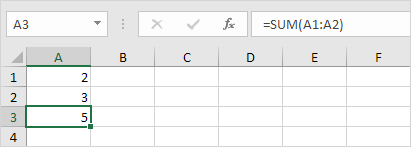### Enter a Formula

To enter a formula, execute the following steps.

1. Select a cell.

2. To let Excel know that you want to enter a formula, type an equal sign (=).

3. For example, type the formula A1+A2.Tip: instead of typing A1 and A2, simply select cell A1 and cell A2.

4. Change the value of cell A1 to 3.

Worked Example:   Highlight unique values in ExcelExcel automatically recalculates the value of cell A3. This is one of Excel’s most powerful features!

### Edit a Formula

When you select a cell, Excel shows the value or formula of the cell in the formula bar.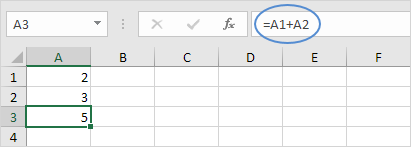1. To edit a formula, click in the formula bar and change the formula.2. Press Enter.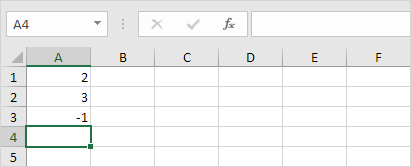### Operator Precedence

Excel uses a default order in which calculations occur. If a part of the formula is in parentheses, that part will be calculated first. It then performs multiplication or division calculations. Once this is complete, Excel will add and subtract the remainder of your formula. See the example below.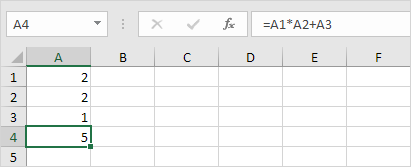First, Excel performs multiplication (A1 * A2). Next, Excel adds the value of cell A3 to this result.

Another example,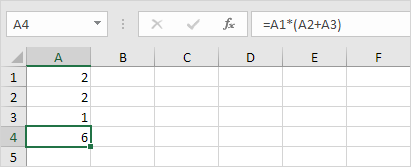First, Excel calculates the part in parentheses (A2+A3). Next, it multiplies this result by the value of cell A1.

### Copy/Paste a Formula

When you copy a formula, Excel automatically adjusts the cell references for each new cell the formula is copied to. To understand this, execute the following steps.

Worked Example:   Floating Point Errors in Excel

1. Enter the formula shown below into cell A4.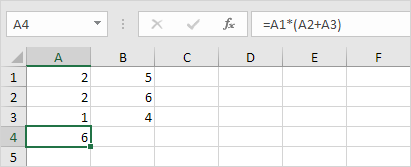2a. Select cell A4, right click, and then click Copy (or press CTRL + c)……next, select cell B4, right click, and then click Paste under ‘Paste Options:’ (or press CTRL + v).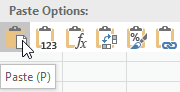2b. You can also drag the formula to cell B4. Select cell A4, click on the lower right corner of cell A4 and drag it across to cell B4. This is much easier and gives the exact same result!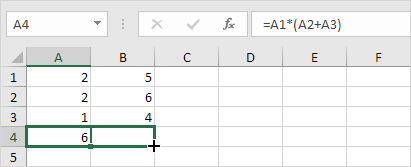Result. The formula in cell B4 references the values in column B.### Insert a Function

Every function has the same structure. For example, SUM(A1:A4). The name of this function is SUM. The part between the brackets (arguments) means we give Excel the range A1:A4 as input. This function adds the values in cells A1, A2, A3 and A4. It’s not easy to remember which function and which arguments to use for each task. Fortunately, the Insert Function feature in Excel helps you with this.

Worked Example:   Understanding Named Range, Named Constant, Name Manager in Excel Formulas

To insert a function, execute the following steps.

1. Select a cell.

2. Click the Insert Function button.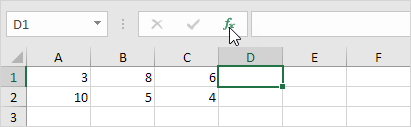The ‘Insert Function’ dialog box appears.

3. Search for a function or select a function from a category. For example, choose COUNTIF from the Statistical category.4. Click OK.

The ‘Function Arguments’ dialog box appears.

5. Click in the Range box and select the range A1:C2.

6. Click in the Criteria box and type >5.

7. Click OK.Result. The COUNTIF function counts the number of cells that are greater than 5.Note: instead of using the Insert Function feature, simply type =COUNTIF(A1:C2,”>5″). When you arrive at: =COUNTIF( instead of typing A1:C2, simply select the range A1:C2.